# teaching vpython to children

I run a small python teaching business in Vienna, Austria teaching the Python programming language. During the summer vacation, I teach every week a new group of children, typically for 15 hours (9:00 a.m. until 12:00 a.m., from Monday until Friday).

As my last group consisted of only two girls and a boy (aged 11 and 12 years old), I decided to experiment a bit and center the whole course around vpython - a python library to create and animate 3D objects. It was not my first attempt to teach vpython to children, but my first time to do so in a summer course setting.

###### click on the images to zoom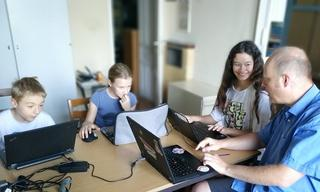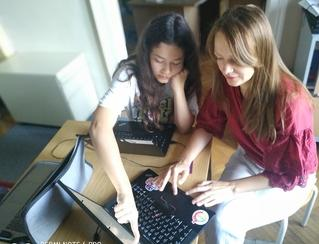image rights (children photos):
(c) 2023 Horst JENS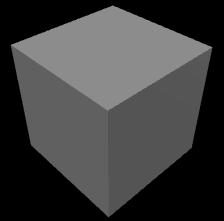the result of the vp.box() command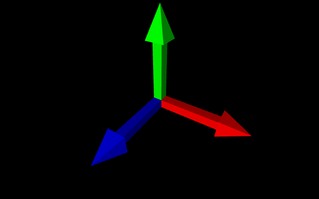3 arrows symbolizing the X, Y, Z -axes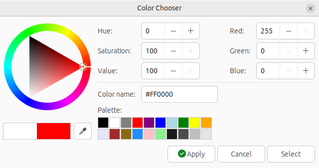geany color chooser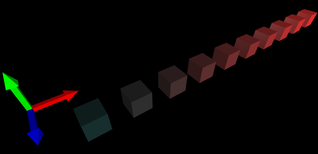a row of cubes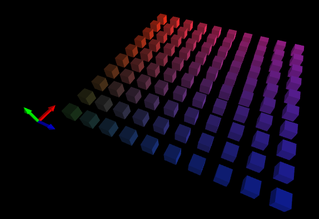a field of cubesa cube of cubes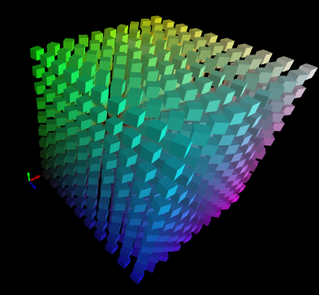a cube of spinning cubes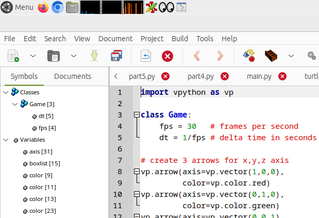a (10 x 10) chessboard made of cubes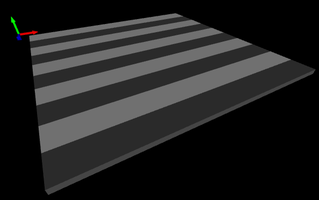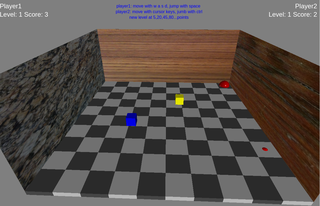game with vpython (1 level)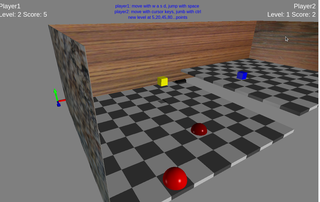game with vpython (2 levels)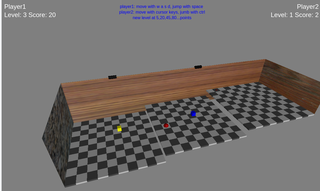game with vpython (3 levels)

The reason to start the course with vpython -instead of a more traditional approach using "pure" python, followed up by some 2d graphic library as turtle graphics- was that all three of my students had extensive experience of playing the three-dimensional game Minecraft and were therefore somewhat familiar with the concept of a three-dimensional space - at least they could recognize what an x,y and z-axis is. Furthermore, two children had some programming experience using Scratch and one girl even had some knowledge of JavaScript.

### Preparations

Austria has recently started to hand out laptops (or tablets) to each 11-year-old student, so I enjoyed the situation that in this course every student could work on it's own laptop. After the necessary installations of Python, Vpython and Geany as a code editor,

I started with a very minimal introduction into the Python programming language using IDLE before switching directly to vpython:

I demonstrated how to create a for-loop and then a nested for-loop.

```>>>for a in range(10):
...     for b in range(10):
...         print(a,"*",b,"=",a*b)
```

In vpython, i started with creating a cube:

```import vpython as vp
vp.box()
```

and continued by trying to explain what a vector is ("some kind of arrow") and referring to the x-axis, y-axis and z-axis that the children already know from playing Minecraft.

```import vpython as vp
# 3 arrows for axis
# x-axis
vp.arrow(axis=vp.vector(1,0,0),
color=vp.vector(1,0,0))
# y-axis
vp.arrow(axis=vp.vector(0,1,0),
color=vp.vector(0,1,0))
# z-axis
vp.arrow(axis=vp.vector(0,0,1),
color=vp.vector(0,0,1))
```

At this point in time I was hampered a bit by the fact that my code editor of choice, Geany, has a very nice color-chooser but displays the Red-Green-Blue value of a color only as hexadecimal value and as tuple three of integers (from 0 to 255). Vpython demands a color value either as a decimal value (from 0.0 to 1.0) so I had some explaining to do.

To practice the understanding of (nested) for-loops I showed my students how to create a row of cubes,a field of cubes and a cube of cubes, each time using the loop variables to change the color:

### a row of cubes

```import vpython as vp

# create 3 arrows for x,y,z axis

vp.arrow(axis=vp.vector(1,0,0),
color=vp.color.red)
vp.arrow(axis=vp.vector(0,1,0),
color=vp.color.green)
vp.arrow(axis=vp.vector(0,0,1),
color=vp.color.blue)

for x in range(1,11):
vp.box(pos=vp.vector(x,0,1),
size=vp.vector(0.5,0.5,0.5),
color = vp.vector(x/10, 0.2,0.2))
```

### a field of cubes

```import vpython as vp

# create 3 arrows for x,y,z axis

vp.arrow(axis=vp.vector(1,0,0),
color=vp.color.red)
vp.arrow(axis=vp.vector(0,1,0),
color=vp.color.green)
vp.arrow(axis=vp.vector(0,0,1),
color=vp.color.blue)

for x in range(1,11):
for z in range(1,11):
vp.box(pos=vp.vector(x,0,z),
size=vp.vector(0.5,0.5,0.5),
color = vp.vector(x/10, 0.2,z/10))
```

### a cube of cubes

```import vpython as vp

# create 3 arrows for x,y,z axis

vp.arrow(axis=vp.vector(1,0,0),
color=vp.color.red)
vp.arrow(axis=vp.vector(0,1,0),
color=vp.color.green)
vp.arrow(axis=vp.vector(0,0,1),
color=vp.color.blue)

for x in range(1,11):
for y in range(1,11):
for z in range(1,11):
vp.box(pos=vp.vector(x,y,z),
size=vp.vector(0.5,0.5,0.5),
color = vp.vector(x/10, y/10,z/10))
```

### cube of spinning cubes

I finished my vpython introduction by making each cube in the "cube of cubes" example spin using a different speed. The result is rather pleasing because of the automatic light-reflecting effects of vpython:

```import vpython as vp

class Game:
fps = 30   # frames per second
dt = 1/fps # delta time in seconds

# create 3 arrows for x,y,z axis
vp.arrow(axis=vp.vector(1,0,0),
color=vp.color.red)
vp.arrow(axis=vp.vector(0,1,0),
color=vp.color.green)
vp.arrow(axis=vp.vector(0,0,1),
color=vp.color.blue)
# list of boxes
boxlist = []
# create 10x10x10 boxes
for x in range(1,11):
for y in range(1,11):
for z in range(1,11):
boxlist.append(
vp.box(pos=vp.vector(x,y,z),
size=vp.vector(0.5,0.5,0.5),
color=vp.vector(x/10,y/10,z/10))
)
# animation: spin each cube using a different speed
while True:
vp.rate(Game.fps) # make only 30 frames per second
rotation_speed = 0.001
for b in boxlist:
b.rotate(angle=rotation_speed*Game.dt,
axis=vp.vector(0,1,0))
rotation_speed += 0.001
```

### teaching

At this time, i introduced a python class not so much to explain object-oriented programming concepts but more as a means to organize global variables.

The geany code editor has a side panel to display a tree-view of classes and variables to ease code navigation, and my goal was to teach my students to navigate inside their code in an efficient and fast way.

As my courses are an introduction to python programming and not part of a school curriculum, I have the luxury of not teaching for a test and my main goal is to keep the students interested in the topic of programming. Over the years of teaching children, I learned (the hard way) that often "less is more" when explaining code:

For most of my students, to get the code running on their own computer (and to learn to correct their own typing errors) is a more urgent need then to listen to my explanations about python commands.

The questions (and the understanding) comes with time.

At this point in time (around the second day of the course) I was more or less satisfied with the typing skills of my students and I was confident that they could reproduce some code lines that I typed on my computer.

As I often saw when teaching coding to children, all the "nerdy" concepts of teaching programming (loops, variables, functions, classes) soon become a side show when the students are challenged with simply typing and navigating inside a text editor.

On the other hand, I feel that the topic of mixing languages (python commands (and most good documentation) is in English, while all my students were native German speakers) is not much of a problem; If i ask what an (English) keyword means in German the students can often give me a reasonable answer, and older students are very enthusiastic to point out any spelling and pronunciation errors of my English.

### chessboard

I continued the course by letting the students use Idle to explore the concept of a remainder and the modulo operator.

Switching back to vpython and geany, we created a chessboard (an array of cubes) using the modulo operator to "paint" every other field with a different color.

As my students discovered, changing the size of the chessboard (9x9 instead 8x8) can result in a pattern of stripes if the code is not flexible enough:

```import vpython as vp

class Game:
fps = 30   # frames per second
dt = 1/fps # delta time in seconds
board_size = 10
dark = vp.vector(0.3,0.3,0.3)
light = vp.vector(0.8,0.8,0.8)

# create 3 arrows for x,y,z axis
vp.arrow(axis=vp.vector(1,0,0),
color=vp.color.red)
vp.arrow(axis=vp.vector(0,1,0),
color=vp.color.green)
vp.arrow(axis=vp.vector(0,0,1),
color=vp.color.blue)

# create chessboard
i = 0
for x in range(1,Game.board_size+1):
remainder = i%2
for z in range(1,Game.board_size+1):
i += 1
if i % 2 == 0:
board_color = Game.dark
else:
board_color = Game.light
vp.box(pos=vp.vector(x,-0.1,z),
size=vp.vector(1,0.2,1),
color=board_color)
# outcomment the next two lines and change
# the board size to see if you get "stripes"
if i%2 == remainder:
i+=1
```

### game

At this point in time during the course, the focus of my teaching changed from explaining generic vpython concepts towards building a game using vpython.

I initially prepared a Minecraft-like concept game where a player can move in 3 dimensions and create "boxes". However my students were quite happy to control a player in two dimension (sliding around the chessboard) and later apply a jump functionality to the player. In the end, we made some kind of 3D - Platform game where a player must collect red balls and try to evade an triangular enemy.

This game-programming occupied the remaining days of the course and kept me busy. My students were mostly busy discussing pros and cons of desired features ("Do we need to collect coins? No, that looks too much like a Mario game...") and I was busy trying to implement the most urgent desired features into the game.

At the end of the workshop we had an somewhat playable version but it took me a full weekend to re-do and improve the code to have an program that had around the same features like the version created during the course but had a more sophisticated structure and cleaner code.

### jumping between chessboards

One particular feature that took me very long to solve in a satisfying way was the the ability of the player to jump from a lower chessboard to a higher chessboard. (Like jumping between platforms in a 2-dimensional platformer game). The code to jump itself was not difficult to create, neither the ability to "stay" on a platform - i did this during the course already with the students.

The difficult part was that the player should not be able to "slide" toward the higher platform, but only be able to "jump" to it. On the other hand, the player should be able to slide from the higher platform towards the lower platform and susequently falling down and landing on the lower platform.

Vpython itself is created for and by physic students but has no built-in physics engine - it's the job of the programmer to transform the law of physics into code.

After much bug-hunting (players stuck on invisible walls, wandering off into the void, teleporting erratic around etc.) I finally produced a version that allowed the player to jump up and slide down between an undefined number of platforms (like a big stair).

### conclusion

Using vpython to teach python to children can be a very rewarding experience: The children are instantly immersed into the world of programming because of the visual pleasing 3D output, while needing to type a minimal number of code lines.

Vpython is also a great way to teach advanced mathematical concepts like 3-dimensional vectors or vector-operations like a cross-product of vectors.

A disadvantage of using vpython with beginners is that the bug-hunting and error-message reading while working with vpython can be difficult: Do to the very complex technology behind vpython, the original error message gets often swamped by several follow-up error messages that are hard to read, specially for beginners.

I can recommend vpython as a tool to teach python to children when the children have some typing / text editing skills and ideally some implicit knowledge of 3d-computer graphics (like from playing Minecraft).

For younger children (<10 years) or children without good typing skills I would advise to explore visual programming systems like Beetleblocks first before diving into vpython.

Contents © 2023 Horst JENS - This work is licensed under a Creative Commons Attribution-ShareAlike 4.0 International License.